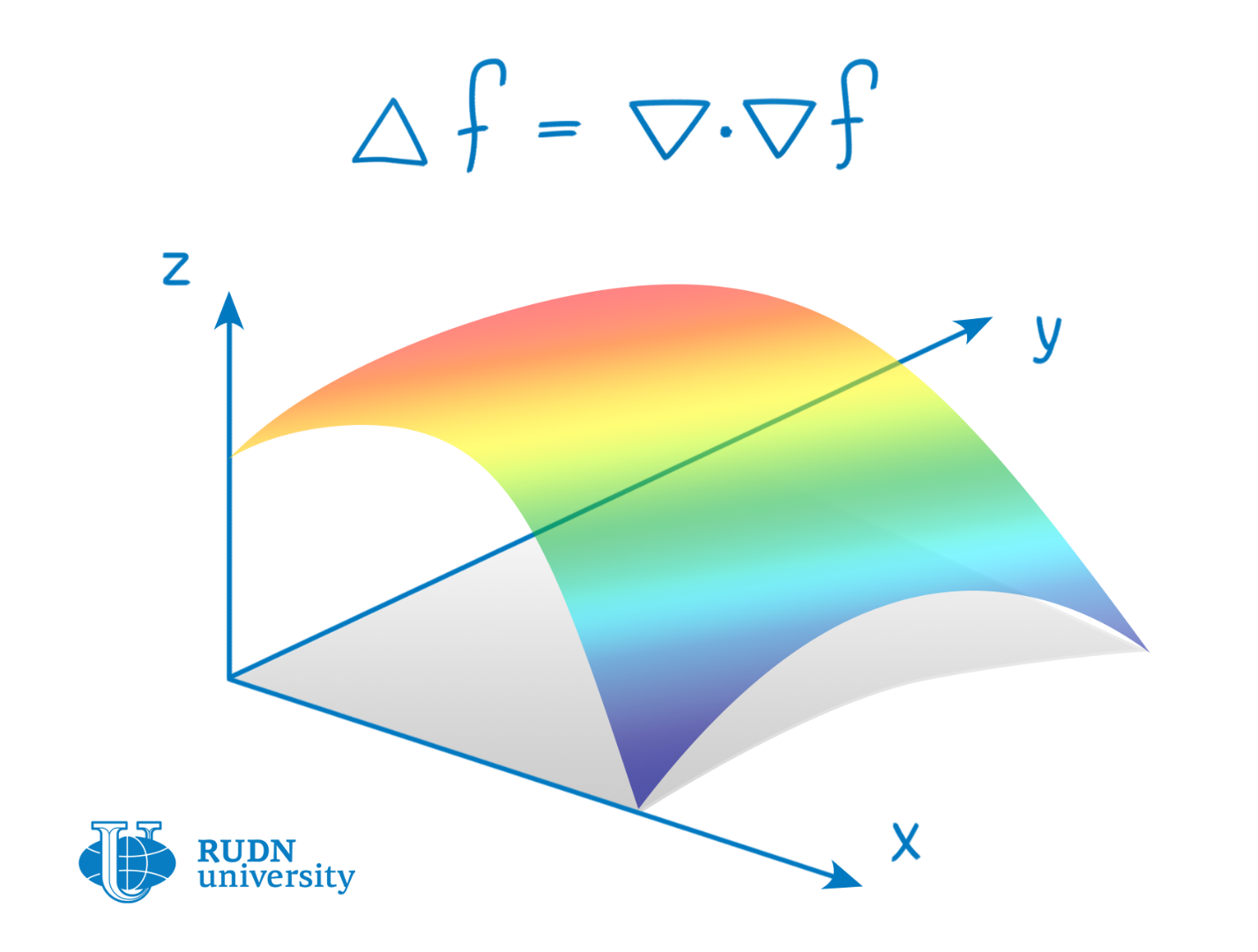RUDN University mathematicians have obtained an estimate of inverse problems of the Laplace differential equation, which allows describing the type of space under study

# RUDN University mathematicians have obtained an estimate of inverse problems of the Laplace differential equation, which allows describing the type of space under study

RUDN mathematicians received an estimate of the solution of inverse problems of the Laplace differential equation. Having a set of values that characterize the Laplace map and the Dirichlet-Neumann limit parameters, they were able to obtain conditions that determine the type of space under study. The obtained result allows us to describe the nature of physical space in the problems of electrostatics, electrodynamics, quantum mechanics, continuum physics and in the problem of studying the thermal conductivity of the medium.RUDN mathematicians received an estimate of the solution of inverse problems of the Laplace differential equation. Having a set of values that characterize the Laplace map and the Dirichlet-Neumann limit parameters, they were able to obtain conditions that determine the type of space under study. The obtained result allows us to describe the nature of physical space in the problems of electrostatics, electrodynamics, quantum mechanics, continuum physics and in the problem of studying the thermal conductivity of the medium.

When solving physical problems using mathematical methods, functions are put in accordance with certain physical processes. For example, to describe the thermal conductivity process, we need the Laplace differential equation-the Laplace map. These functions operate within a specific characteristic space that corresponds to the physical model of the phenomenon in question.

Experimental data of a physical problem can be a set of values that characterize the type of Laplace mapping for a specific space. Direct problems of the differential equation allow us to obtain this set of characteristic values in the known mathematical form of the Laplace map. In this case, the solution of inverse problems of these equations for a known set of characteristic values allows us to study the mathematical form of space, and therefore the physical process of the problem under study.

The space is characterized by a metric-a rule for distributing elements in this space. Knowing the mathematical form of the space metric, you can get the space structure of the physical model of the problem under study. Thus, by analyzing neural activity data (characteristic values), you can get a metric of the neural network space that will be associated with the physical model of this network.

It is known that the type of metric can be uniquely defined up to the level at which two metrics ― g1 and g2 ― become equivalent for a slightly different set of characteristic values, so the solution will not be the only one. However, if there are two metrics with the same set of characteristic values, a conversion is allowed that converts one metric to another.

For an adequate description of the type of space under study, it is necessary to make sure that the found metric type is correct when solving a direct problem of differential equations. For such verification, a tool can be used to study the uniqueness of the solution of inverse problems on an arbitrarily bounded area of space.

After considering a given set of characteristic values on an arbitrarily fixed domain, the authors obtained a mathematical estimation of the uniqueness of the solution for the inverse problem in determining the metric of the Laplace operator.

Another type of input for the inverse problem can be boundary conditions under which the behavior of the physical model is known. In this case, the authors used data on Dirichlet-Neumann boundary conditions for Laplace mapping on an arbitrary region of the studied space to obtain an estimate of the uniqueness of the solution of the inverse problem.

Article in the journal Inverse Problems & Imaging.

Student's Scientific Initiatives View all
03 Nov 2017
June 22 - 26, 2017 in Barnaul, Altai State University, took place the Summer Academy of the BRICS Youth Assembly, an international event that brought together representatives of different countries
1366
Scientific Conferences View all
03 Nov 2017
RUDN University organized the first 5G Summit R&D Russia on June 19 - 20, 2017
1719
20 Apr
RUDN University agronomists increased wheat yield by 65%

RUDN University agronomists have proposed a new scheme for fertilizing winter wheat, which allows increasing the yield by 68%. The key to this is in the combination of nitrogen and growth regulators.

56
20 Apr
RUDN Biologists Study Live Microorganisms in Toxic Liquids for Metalworking

RUDN biologists have studied microorganisms that can survive in metalworking fluids. The results will allow “picking up” bacteria and fungi that can process toxic waste fluids into a harmless product.

55
20 Apr
The chemist RUDN created a catalyst for more efficient oxidation of cyclohexane

The chemist of RUDN together with colleagues from Iran and Spain created a catalyst based on palladium and nickel for the oxidation of cyclohesane in the production of adipic acid, which is used for the production of cleaning products, food dyes and other substances. The new catalyst made it possible to double the consumption of cyclohexane.

45